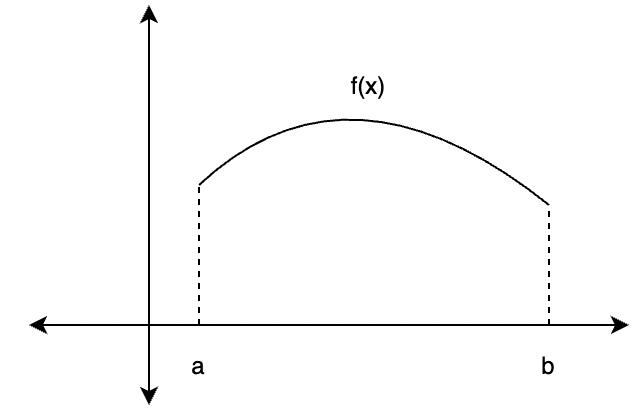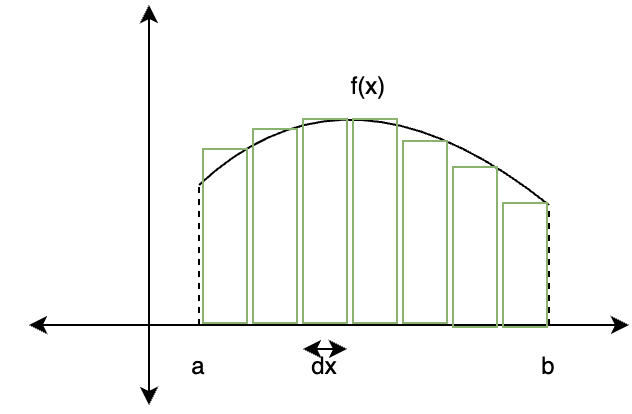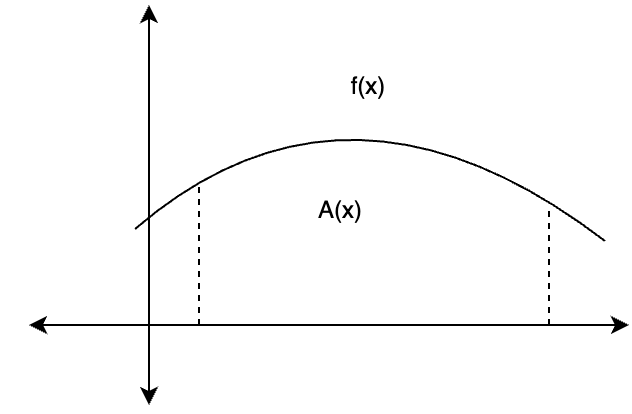GFG App
Open AppBrowser
Continue

Differential calculus is centered on the concept of derivative, the derivatives measure the rate of change for the different values of the function. Geometrically speaking, they are the slope of the tangent at any point. Integrals are the reverse process of differentiation, integrals are motivated by the sum, just like derivatives calculate the difference, integrals calculate the sum for different functions, now this summation can be of anything. Mostly it is used for calculating the area and volumes of arbitrary shapes. Let’s look at these concepts in detail.

### Introduction to Integrals

Consider a function “f”, which is differentiable in the interval “I” which means that its derivative exists at all the points in the interval I. Now, the question that comes to mind is, if the derivative of the function at each point is given, is it possible to generate the function back again? The function will be called anti-derivative. The process through which we generate these functions are called integration and the formula that gives these anti-derivatives is called an indefinite integral.

Integrals as the inverse of differentiation

Consider derivatives of some functions given below,In the above equations, consider, here x2 is the anti-derivative for the function f'(x) = 2x. This follows for the above derivatives too.

Note that if these functions are added with constants, there is no difference to the derivatives as the derivative of a constant is zero.So, it can be concluded that for any function, it’s anti-derivatives are infinite. For example, for the function f(x), let it’s anti-derivative be F(x),denotes the integration. This will represent the integration operation over any function. The table below represents the symbols and meanings related to integrals.

### Properties and Integral of some Standard Functions

We come across a lot of functions every day, and it becomes essential to learn the properties of integrals to simplify the process of integration. It is also necessary that integrals of some standard functions are memorized so that it becomes easier to solve the complex integrals which are usually made up of some elementary simple functions. The table below lists down some integrals of standard functions.

Properties of Integrals:

Property 1:Property 2:Property 3:### Geometrical Interpretation of Integrals

Integrals are usually used for calculating the areas under the curve. Although there are formulas available, they are only available for standard shapes. Often arbitrarily complex shapes are encountered, and it is not possible to develop and remember a formula for every shape. Thus, integrals help in generalizing the method for calculating the areas and the volume. Consider a function f(x), the objective is to calculate the area of the function.The function is divided up into different parts in the shape of rectangles, these parts add up and form the area. The width of each rectangle isAs the number of rectangles increase, the width of these rectangles becomes very small and can be denoted by “dx”. The area of each rectangle becomes f(x)dx. The total area is the sum of areas of all these small rectangles,Let’s look at some sample problems

### Sample Problems

Question 1: Find the integral for the given function f(x),

f(x) = 2sin(x) + 1

Solution:

Given f(x) = 2sin(x) + 1

sin(x) is a standard function, and its integral is known.Using the property 2 mentioned above,Question 2: Find the integral for the given function f(x),

f(x) = 2x2 + 3x

Solution:

Given f(x) =2x2 + 3x

2x2 + 3x is a polynomial function, and it can be broken down in different parts.Using the property 1 mentioned above,Question 3: Find the integral for the given function f(x),

f(x) = x3 + 3x2 + x + 1

Solution:

Given f(x) = x3 + 3x2 + x + 1

x3 + 3x2 + x + 1 is a polynomial function, and it can be broken down in different parts.Using the property 1 mentioned above,Question 4: Find the integral for the given function f(x),

f(x) = 5x-2 + x + 1

Solution:

Given f(x) = 5x-2 + x + 1

Using the reverse power ruleUsing the property 1 mentioned above,Question 5: Find the integral for the given function f(x),

f(x) = sin(x) + 5cos(x)

Solution:

Given f(x) = sin(x) + 5cos(x)

sin(x) and cos(x) are standard functions, and its integral is known.Using the properties 1 and 2 mentioned above,Question 6: Find the integral for the given function f(x),

f(x) = 5x-2 + x4 + x

Solution:

Given f(x) = 5x-2 + x4 + x

Using the reverse power ruleUsing the properties 1 and 2 and power rule mentioned above,Question 7: Find the integral for the given function f(x),

f(x) = 5x-1 + 12

Solution:

Given f(x) = 5x-1 + 12

Using the reverse power ruleUsing the properties 1 and 2My Personal Notes arrow_drop_up